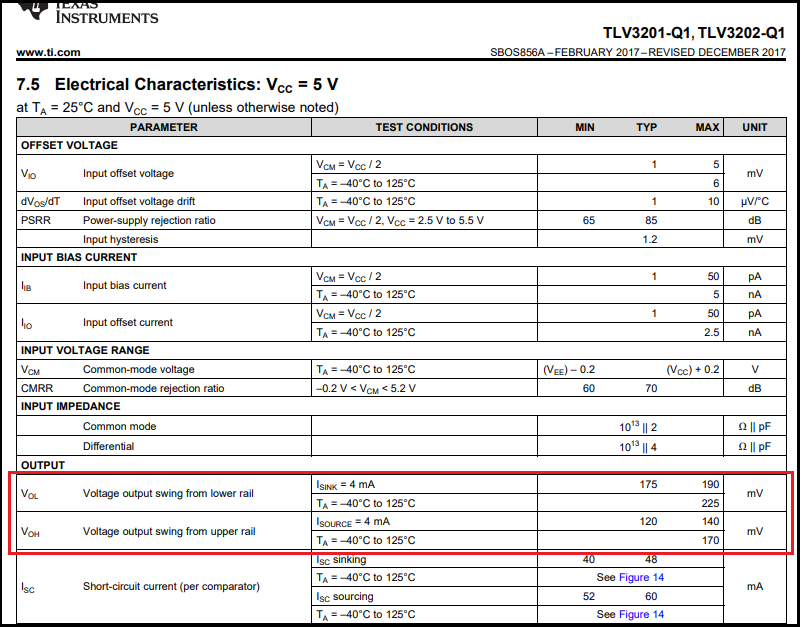If you have a related question, please click the "Ask a related question" button in the top right corner. The newly created question will be automatically linked to this question.

# TLV3201-Q1: Temperature curve

Part Number: TLV3201-Q1
Other Parts Discussed in Thread: TLV3201

Hi I have read the datasheet of TLV3201-Q1,

There has the curve of Output Voltage vs Output Current with full range temperature of 2.7V and 5.5V

but we are using 5V as the input voltage with tolerance ±1%,

so can TI offer the curve of Output Voltage vs Output Current with full range temperature of 5V±1%?

or the calculate between Vout with Temperature and Current

We are using as frequency oscillator, so the vout voltage will effect the RC time constant

Thanks for apply.• Hi,

all the needed information is on page 5 of datasheet:For small output currents the output of comparator looks like a resistor being connected to the supply rails. So you get a 120mV / 4mA = 30R "resistor" to the positive supply voltage and a 175mV / 4mA = 43.8R "resistor" to the negative supply voltage (signal ground). And you can even calculate the maximum resistances with changing ambient temperature :-)

Kai

• Hello,

Any data we would have would have been used to create the graphs you show. As Kai showed and explained, the tables show the limits over temperature. The difference between 5V and 5.5V is minimal.

The best way to minimize the effects of the output swing is to minimize the loading of the output. Peak currents will flow at the transitions as the cap first charges and discharges. R1 will set the peak current (5V/6.8k = 735uA).

So to conserve the output swing, you could bump the resistor values up by a decade - particularly the R2/R3/R4 divider network (say, 100k each). Also consider any loads the output would be driving. If the load may be changing, you may wish to place a buffer logic gate on the output.

• Hi Paul,

Thanks for apply, and I have another question,

Does TI have the simplis model of TLV3201-Q1?

I have only seen the TI-NA model

Thanks!

• Hello,

I see samples available. Click on the Request sample link in the Ordering & quality tab of the product folder.

• Hi Paul,

I have seen the model of TLV3201, but it's not automotive series,

is that mean that TLV3201-Q1 and TLV3201 parameter are the same?

Thanks for apply

• Hello,

Yes. The models only model typical behavior, and the typicals are the same between the two devices, so the same model can be used for both.

If there was a model for the Q1, it would be the same core model, but simply renamed with -Q1.